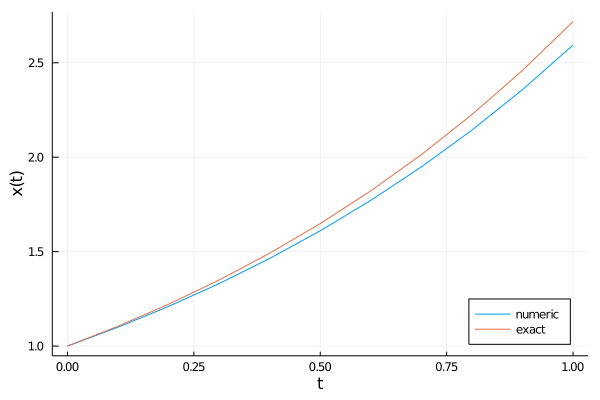dummy-link

26

4

5

5

# GeometricIntegrators.jl

Julia library of geometric integrators for differential equations.

GeometricIntegrators.jl is a library of geometric integrators for ordinary differential equations, stochastic differential equations and differential algebraic equations in Julia. Its main aim is the democratization and proliferation of geometric integrators, providing a comprehensive collection of standard and structure-preserving algorithms under a unified interface. Furthermore it serves as testbed for the implementation and verification of novel geometric integrators, in particular their analysis with respect to long-time stability and conservation of geometric structures. GeometricIntegrators.jl can be used either interactively or as computational core in other codes. It provides both, a high-level interface that requires only very few lines of code to solve an actual problem, and a lean low-level interface that allows for straightforward integration into application codes via the exchange of very small data structures. Due to the modular structure and the use of the multiple dispatch paradigm, the library can easily be extended, e.g., towards new algorithms or new types of equations. GeometricIntegrators.jl is designed to minimize overhead and maximize performance in order to be able to perform simulations with millions or even billions of time steps to facilitate the study of the long-time behaviour of both numerical algorithms and dynamical systems.

## Installation

GeometricIntegrators.jl and all of its dependencies can be installed via the Julia REPL by typing

``````]add GeometricIntegrators
``````

## Basic usage

In the simplest cases, the use of GeometricIntegrators.jl requires the construction of two objects, an equation and an integrator. For many standard methods, the integrator can be implicitly selected by specifying an equation and a tableau.

Before any use, we need to load `GeometricIntegrators`,

``````using GeometricIntegrators
``````

Then we can create an `ODE` object for the equation ẋ(t) = x(t) with initial condition x(0)=1 by

``````ode = ODE((t, x, ẋ) -> ẋ = x, [1.0]);
``````

An integrator for this ODE, using the tableau for the explicit Euler method and a time step of Δt=0.1, is obtained by

``````int = Integrator(ode, TableauExplicitEuler(), 0.1);
``````

With that, the solution for nₜ=10 time steps is simply computed by

``````sol = integrate(ode, int, 10);
``````

which returns an object holding the solution for all time steps. With the help of the Plots.jl package we can visualise the result and compare with the exact solution:

``````using Plots
plot(xlims=[0,1], xlab="t", ylab="x(t)", legend=:bottomright)
plot!(sol.t, sol.q[1,:], label="numeric")
plot!(sol.t, exp.(sol.t), label="exact")
``````## References

If you use GeometricIntegrators.jl in your work, please consider citing it by

``````@misc{Kraus:2020:GeometricIntegrators,
title={GeometricIntegrators.jl: Geometric Numerical Integration in Julia},
author={Kraus, Michael},
year={2020},
howpublished={\url{https://github.com/JuliaGNI/GeometricIntegrators.jl}},
doi={10.5281/zenodo.3648325}
}
``````

08/22/2016

28 days ago

1974 commits